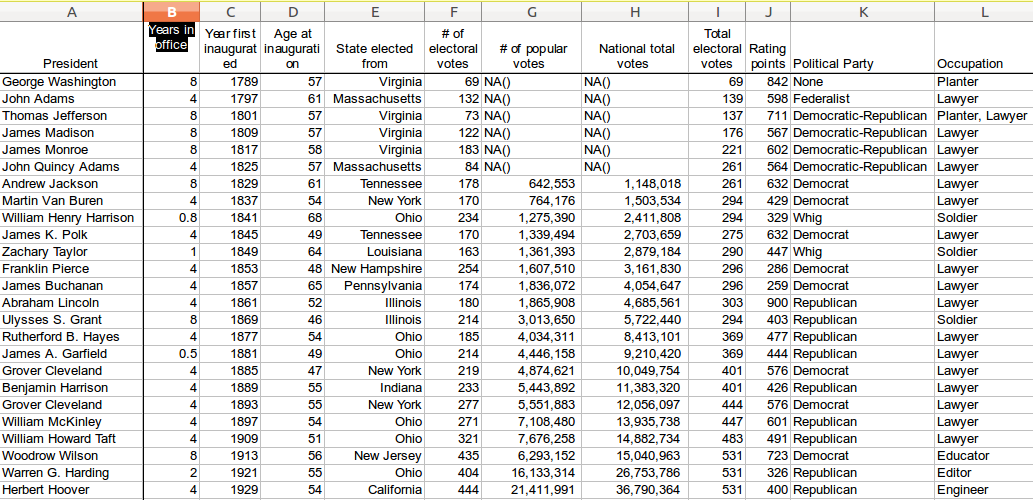Python hosting: Host, run, and code Python in the cloud!

You can use pandas to read data from an Excel file into a DataFrame, and then work with the data just like you would any other dataset.

To read an Excel file into a DataFrame using pandas, you can use the read_excel() function. The read_excel() function returns a DataFrame by default, so you can access the data in your DataFrame using standard indexing and slicing operations.

Pandas, a data analysis library, has native support for loading excel data (xls and xlsx). The method read_excel loads xls data into a Pandas dataframe:

If you have a large excel file you may want to specify the sheet:

Related course
Data Analysis with Python Pandas

The code below reads excel data into a Python dataset (the dataset can be saved below).

The dataframe can be used, as shown in the example below:

Dataset
For purpose of demonstration, you can use the dataset from: depaul.edu.A large dataset stored in XLS format